# Mean State

Period Mean (original grids) [W/m2]
Model Period Mean (intersection) [W/m2]
Model Period Mean (complement) [W/m2]
Benchmark Period Mean (intersection) [W/m2]
Benchmark Period Mean (complement) [W/m2]
Bias [W/m2]
RMSE [W/m2]
Phase Shift [months]
Bias Score 
RMSE Score 
Seasonal Cycle Score 
Spatial Distribution Score 
Interannual Variability Score 
Overall Score 
Benchmark [-] 187.
CLM4 [-] 181. 181. 0.00 186. 206. -5.09 15.5 0.746 0.71 0.50 0.91 0.92 0.81 0.72
CLM4.5 [-] 181. 181. 0.00 186. 206. -4.81 15.3 0.744 0.72 0.50 0.91 0.93 0.81 0.73
CLM5 [-] 182. 182. 0.00 186. 206. -4.45 15.1 0.823 0.73 0.49 0.90 0.94 0.80 0.73
Period Mean (original grids) [W/m2]
Model Period Mean (intersection) [W/m2]
Model Period Mean (complement) [W/m2]
Benchmark Period Mean (intersection) [W/m2]
Benchmark Period Mean (complement) [W/m2]
Bias [W/m2]
RMSE [W/m2]
Phase Shift [months]
Bias Score 
RMSE Score 
Seasonal Cycle Score 
Spatial Distribution Score 
Interannual Variability Score 
Overall Score 
Benchmark [-] 198.
CLM4 [-] 193. 193. 0.00 198. 214. -4.63 14.4 0.711 0.70 0.52 0.92 0.98 0.86 0.75
CLM4.5 [-] 194. 194. 0.00 198. 214. -3.67 14.3 0.718 0.71 0.52 0.92 0.98 0.86 0.75
CLM5 [-] 195. 195. 0.00 198. 214. -2.80 14.2 0.767 0.71 0.52 0.91 0.96 0.85 0.74
Period Mean (original grids) [W/m2]
Model Period Mean (intersection) [W/m2]
Model Period Mean (complement) [W/m2]
Benchmark Period Mean (intersection) [W/m2]
Benchmark Period Mean (complement) [W/m2]
Bias [W/m2]
RMSE [W/m2]
Phase Shift [months]
Bias Score 
RMSE Score 
Seasonal Cycle Score 
Spatial Distribution Score 
Interannual Variability Score 
Overall Score 
Benchmark [-] 199.
CLM4 [-] 195. 195. 0.00 198. 219. -3.69 15.6 0.757 0.80 0.57 0.90 0.99 0.75 0.76
CLM4.5 [-] 196. 196. 0.00 198. 219. -3.23 15.5 0.742 0.80 0.57 0.90 0.99 0.75 0.76
CLM5 [-] 196. 196. 0.00 198. 219. -2.87 15.6 0.787 0.80 0.57 0.89 1.0 0.75 0.76
Period Mean (original grids) [W/m2]
Model Period Mean (intersection) [W/m2]
Model Period Mean (complement) [W/m2]
Benchmark Period Mean (intersection) [W/m2]
Benchmark Period Mean (complement) [W/m2]
Bias [W/m2]
RMSE [W/m2]
Phase Shift [months]
Bias Score 
RMSE Score 
Seasonal Cycle Score 
Spatial Distribution Score 
Interannual Variability Score 
Overall Score 
Benchmark [-] 70.7
CLM4 [-] 66.9 67.0 0.00 70.5 72.8 -3.66 20.9 0.355 0.91 0.74 0.98 1.0 0.80 0.86
CLM4.5 [-] 71.7 71.9 0.00 70.5 72.8 0.485 18.9 0.332 0.91 0.76 0.98 0.99 0.75 0.86
CLM5 [-] 73.1 73.3 0.00 70.5 72.8 1.86 18.4 0.331 0.91 0.77 0.98 1.0 0.74 0.86
Period Mean (original grids) [W/m2]
Model Period Mean (intersection) [W/m2]
Model Period Mean (complement) [W/m2]
Benchmark Period Mean (intersection) [W/m2]
Benchmark Period Mean (complement) [W/m2]
Bias [W/m2]
RMSE [W/m2]
Phase Shift [months]
Bias Score 
RMSE Score 
Seasonal Cycle Score 
Spatial Distribution Score 
Interannual Variability Score 
Overall Score 
Benchmark [-] 194.
CLM4 [-] 175. 175. 0.00 190. 207. -11.0 19.0 1.28 0.55 0.44 0.80 0.96 0.77 0.66
CLM4.5 [-] 175. 175. 0.00 190. 207. -10.9 19.0 1.24 0.55 0.44 0.80 0.96 0.77 0.66
CLM5 [-] 175. 175. 0.00 190. 207. -11.2 19.1 1.25 0.54 0.44 0.80 0.96 0.77 0.66
Period Mean (original grids) [W/m2]
Model Period Mean (intersection) [W/m2]
Model Period Mean (complement) [W/m2]
Benchmark Period Mean (intersection) [W/m2]
Benchmark Period Mean (complement) [W/m2]
Bias [W/m2]
RMSE [W/m2]
Phase Shift [months]
Bias Score 
RMSE Score 
Seasonal Cycle Score 
Spatial Distribution Score 
Interannual Variability Score 
Overall Score 
Benchmark [-] 97.8
CLM4 [-] 102. 102. 0.00 97.6 118. 4.49 15.6 0.227 0.91 0.82 0.98 1.0 0.69 0.87
CLM4.5 [-] 99.6 99.6 0.00 97.6 118. 2.33 16.3 0.232 0.92 0.81 0.98 1.0 0.69 0.87
CLM5 [-] 100. 100. 0.00 97.6 118. 2.99 15.5 0.231 0.92 0.82 0.98 1.0 0.69 0.87
Period Mean (original grids) [W/m2]
Model Period Mean (intersection) [W/m2]
Model Period Mean (complement) [W/m2]
Benchmark Period Mean (intersection) [W/m2]
Benchmark Period Mean (complement) [W/m2]
Bias [W/m2]
RMSE [W/m2]
Phase Shift [months]
Bias Score 
RMSE Score 
Seasonal Cycle Score 
Spatial Distribution Score 
Interannual Variability Score 
Overall Score 
Benchmark [-] 139.
CLM4 [-] 135. 134. 0.00 138. 149. -3.52 16.8 0.371 0.87 0.76 0.97 1.0 0.67 0.84
CLM4.5 [-] 134. 133. 0.00 138. 149. -4.24 16.5 0.344 0.87 0.77 0.97 1.0 0.66 0.84
CLM5 [-] 135. 135. 0.00 138. 149. -2.61 15.9 0.340 0.88 0.77 0.97 1.0 0.67 0.84
Period Mean (original grids) [W/m2]
Model Period Mean (intersection) [W/m2]
Model Period Mean (complement) [W/m2]
Benchmark Period Mean (intersection) [W/m2]
Benchmark Period Mean (complement) [W/m2]
Bias [W/m2]
RMSE [W/m2]
Phase Shift [months]
Bias Score 
RMSE Score 
Seasonal Cycle Score 
Spatial Distribution Score 
Interannual Variability Score 
Overall Score 
Benchmark [-] 181.
CLM4 [-] 173. 173. 0.00 179. 205. -4.55 18.4 0.346 0.76 0.61 0.97 0.97 0.73 0.78
CLM4.5 [-] 174. 173. 0.00 179. 205. -4.18 18.3 0.342 0.76 0.61 0.97 0.96 0.72 0.77
CLM5 [-] 174. 174. 0.00 179. 205. -3.72 18.1 0.320 0.77 0.61 0.97 0.94 0.72 0.77
Period Mean (original grids) [W/m2]
Model Period Mean (intersection) [W/m2]
Model Period Mean (complement) [W/m2]
Benchmark Period Mean (intersection) [W/m2]
Benchmark Period Mean (complement) [W/m2]
Bias [W/m2]
RMSE [W/m2]
Phase Shift [months]
Bias Score 
RMSE Score 
Seasonal Cycle Score 
Spatial Distribution Score 
Interannual Variability Score 
Overall Score 
Benchmark [-] 62.8
CLM4 [-] 59.4 59.8 0.00 63.2 54.4 -4.15 18.4 0.210 0.92 0.76 0.99 0.99 0.79 0.87
CLM4.5 [-] 62.0 62.3 0.00 63.2 54.4 -1.89 16.8 0.165 0.93 0.78 0.99 0.99 0.75 0.87
CLM5 [-] 64.7 65.0 0.00 63.2 54.4 0.684 15.6 0.199 0.93 0.79 0.99 0.99 0.75 0.87
Period Mean (original grids) [W/m2]
Model Period Mean (intersection) [W/m2]
Model Period Mean (complement) [W/m2]
Benchmark Period Mean (intersection) [W/m2]
Benchmark Period Mean (complement) [W/m2]
Bias [W/m2]
RMSE [W/m2]
Phase Shift [months]
Bias Score 
RMSE Score 
Seasonal Cycle Score 
Spatial Distribution Score 
Interannual Variability Score 
Overall Score 
Benchmark [-] 198.
CLM4 [-] 191. 191. 0.00 198. 197. -8.80 16.0 0.341 0.83 0.77 0.97 1.0 0.72 0.84
CLM4.5 [-] 191. 191. 0.00 198. 197. -8.85 16.1 0.334 0.82 0.77 0.98 1.0 0.72 0.84
CLM5 [-] 192. 192. 0.00 198. 197. -7.59 15.6 0.385 0.85 0.76 0.97 1.0 0.72 0.84
Period Mean (original grids) [W/m2]
Model Period Mean (intersection) [W/m2]
Model Period Mean (complement) [W/m2]
Benchmark Period Mean (intersection) [W/m2]
Benchmark Period Mean (complement) [W/m2]
Bias [W/m2]
RMSE [W/m2]
Phase Shift [months]
Bias Score 
RMSE Score 
Seasonal Cycle Score 
Spatial Distribution Score 
Interannual Variability Score 
Overall Score 
Benchmark [-] 120.
CLM4 [-] 112. 112. 0.00 118. 145. -3.16 14.9 0.242 0.92 0.82 0.98 1.0 0.68 0.87
CLM4.5 [-] 113. 113. 0.00 118. 145. -2.25 13.7 0.229 0.92 0.84 0.98 0.99 0.67 0.87
CLM5 [-] 113. 113. 0.00 118. 145. -2.30 13.6 0.227 0.92 0.84 0.98 0.99 0.66 0.87
Period Mean (original grids) [W/m2]
Model Period Mean (intersection) [W/m2]
Model Period Mean (complement) [W/m2]
Benchmark Period Mean (intersection) [W/m2]
Benchmark Period Mean (complement) [W/m2]
Bias [W/m2]
RMSE [W/m2]
Phase Shift [months]
Bias Score 
RMSE Score 
Seasonal Cycle Score 
Spatial Distribution Score 
Interannual Variability Score 
Overall Score 
Benchmark [-] 163.
CLM4 [-] 135. 135. 0.00 142. 173. -5.68 16.9 0.391 0.80 0.70 0.96 1.0 0.71 0.81
CLM4.5 [-] 136. 136. 0.00 142. 173. -5.10 16.2 0.383 0.81 0.71 0.96 1.0 0.72 0.82
CLM5 [-] 137. 137. 0.00 142. 173. -4.59 16.0 0.394 0.82 0.70 0.96 1.0 0.71 0.82
Period Mean (original grids) [W/m2]
Model Period Mean (intersection) [W/m2]
Model Period Mean (complement) [W/m2]
Benchmark Period Mean (intersection) [W/m2]
Benchmark Period Mean (complement) [W/m2]
Bias [W/m2]
RMSE [W/m2]
Phase Shift [months]
Bias Score 
RMSE Score 
Seasonal Cycle Score 
Spatial Distribution Score 
Interannual Variability Score 
Overall Score 
Benchmark [-] 177.
CLM4 [-] 160. 160. 0.00 175. 217. -13.9 18.9 0.288 0.69 0.77 0.98 0.96 0.67 0.81
CLM4.5 [-] 161. 161. 0.00 175. 217. -13.3 18.5 0.287 0.69 0.77 0.98 0.96 0.67 0.81
CLM5 [-] 162. 162. 0.00 175. 217. -12.0 18.0 0.296 0.71 0.76 0.98 0.96 0.67 0.81
Period Mean (original grids) [W/m2]
Model Period Mean (intersection) [W/m2]
Model Period Mean (complement) [W/m2]
Benchmark Period Mean (intersection) [W/m2]
Benchmark Period Mean (complement) [W/m2]
Bias [W/m2]
RMSE [W/m2]
Phase Shift [months]
Bias Score 
RMSE Score 
Seasonal Cycle Score 
Spatial Distribution Score 
Interannual Variability Score 
Overall Score 
Benchmark [-] 69.7
CLM4 [-] 67.6 67.5 0.00 69.6 71.1 -3.27 18.6 0.120 0.91 0.77 0.99 0.98 0.78 0.87
CLM4.5 [-] 70.6 70.5 0.00 69.6 71.1 -0.637 16.9 0.103 0.92 0.78 0.99 0.99 0.76 0.87
CLM5 [-] 70.7 70.6 0.00 69.6 71.1 -0.616 17.1 0.0938 0.92 0.78 0.99 0.98 0.75 0.87
Period Mean (original grids) [W/m2]
Model Period Mean (intersection) [W/m2]
Model Period Mean (complement) [W/m2]
Benchmark Period Mean (intersection) [W/m2]
Benchmark Period Mean (complement) [W/m2]
Bias [W/m2]
RMSE [W/m2]
Phase Shift [months]
Bias Score 
RMSE Score 
Seasonal Cycle Score 
Spatial Distribution Score 
Interannual Variability Score 
Overall Score 
Benchmark [-] 204.
CLM4 [-] 191. 192. 0.00 201. 219. -6.78 16.9 0.484 0.76 0.68 0.94 0.99 0.75 0.80
CLM4.5 [-] 191. 191. 0.00 201. 219. -7.27 17.0 0.495 0.76 0.68 0.94 0.99 0.76 0.80
CLM5 [-] 192. 192. 0.00 201. 219. -6.91 16.8 0.470 0.77 0.68 0.94 0.99 0.75 0.80
Period Mean (original grids) [W/m2]
Model Period Mean (intersection) [W/m2]
Model Period Mean (complement) [W/m2]
Benchmark Period Mean (intersection) [W/m2]
Benchmark Period Mean (complement) [W/m2]
Bias [W/m2]
RMSE [W/m2]
Phase Shift [months]
Bias Score 
RMSE Score 
Seasonal Cycle Score 
Spatial Distribution Score 
Interannual Variability Score 
Overall Score 
Benchmark [-] 150.
CLM4 [-] 146. 146. 0.00 148. 192. -0.358 14.0 0.269 0.93 0.80 0.98 0.98 0.69 0.86
CLM4.5 [-] 145. 145. 0.00 148. 192. -1.23 13.6 0.275 0.94 0.80 0.98 0.99 0.70 0.87
CLM5 [-] 145. 145. 0.00 148. 192. -2.06 13.6 0.274 0.94 0.80 0.98 0.99 0.70 0.87
Period Mean (original grids) [W/m2]
Model Period Mean (intersection) [W/m2]
Model Period Mean (complement) [W/m2]
Benchmark Period Mean (intersection) [W/m2]
Benchmark Period Mean (complement) [W/m2]
Bias [W/m2]
RMSE [W/m2]
Phase Shift [months]
Bias Score 
RMSE Score 
Seasonal Cycle Score 
Spatial Distribution Score 
Interannual Variability Score 
Overall Score 
Benchmark [-] 110.
CLM4 [-] 111. 111. 0.00 110. 117. 1.62 14.4 0.121 0.92 0.83 0.99 0.98 0.69 0.87
CLM4.5 [-] 112. 112. 0.00 110. 117. 2.22 14.0 0.115 0.92 0.84 0.99 1.0 0.67 0.88
CLM5 [-] 111. 111. 0.00 110. 117. 1.63 13.7 0.114 0.93 0.84 0.99 1.0 0.68 0.88
Period Mean (original grids) [W/m2]
Model Period Mean (intersection) [W/m2]
Model Period Mean (complement) [W/m2]
Benchmark Period Mean (intersection) [W/m2]
Benchmark Period Mean (complement) [W/m2]
Bias [W/m2]
RMSE [W/m2]
Phase Shift [months]
Bias Score 
RMSE Score 
Seasonal Cycle Score 
Spatial Distribution Score 
Interannual Variability Score 
Overall Score 
Benchmark [-] 161.
CLM4 [-] 158. 158. 0.00 161. 163. -2.90 15.3 0.193 0.92 0.82 0.99 0.98 0.69 0.87
CLM4.5 [-] 157. 157. 0.00 161. 163. -3.91 14.7 0.186 0.91 0.82 0.99 0.99 0.71 0.87
CLM5 [-] 159. 159. 0.00 161. 163. -1.43 14.3 0.202 0.93 0.82 0.99 0.98 0.70 0.87
Period Mean (original grids) [W/m2]
Model Period Mean (intersection) [W/m2]
Model Period Mean (complement) [W/m2]
Benchmark Period Mean (intersection) [W/m2]
Benchmark Period Mean (complement) [W/m2]
Bias [W/m2]
RMSE [W/m2]
Phase Shift [months]
Bias Score 
RMSE Score 
Seasonal Cycle Score 
Spatial Distribution Score 
Interannual Variability Score 
Overall Score 
Benchmark [-] 179.
CLM4 [-] 173. 173. 0.00 178. 218. -3.73 14.4 0.787 0.72 0.45 0.90 0.96 0.80 0.72
CLM4.5 [-] 173. 173. 0.00 178. 218. -3.54 14.4 0.787 0.73 0.45 0.90 0.96 0.81 0.72
CLM5 [-] 173. 173. 0.00 178. 218. -3.71 14.5 0.798 0.73 0.45 0.90 0.96 0.80 0.71
Period Mean (original grids) [W/m2]
Model Period Mean (intersection) [W/m2]
Model Period Mean (complement) [W/m2]
Benchmark Period Mean (intersection) [W/m2]
Benchmark Period Mean (complement) [W/m2]
Bias [W/m2]
RMSE [W/m2]
Phase Shift [months]
Bias Score 
RMSE Score 
Seasonal Cycle Score 
Spatial Distribution Score 
Interannual Variability Score 
Overall Score 
Benchmark [-] 185.
CLM4 [-] 180. 181. 0.00 184. 202. -3.68 15.7 0.679 0.82 0.64 0.92 0.95 0.72 0.78
CLM4.5 [-] 180. 180. 0.00 184. 202. -3.85 15.8 0.646 0.82 0.64 0.92 0.96 0.72 0.78
CLM5 [-] 180. 180. 0.00 184. 202. -4.33 15.8 0.702 0.82 0.64 0.91 0.97 0.72 0.78
Period Mean (original grids) [W/m2]
Model Period Mean (intersection) [W/m2]
Model Period Mean (complement) [W/m2]
Benchmark Period Mean (intersection) [W/m2]
Benchmark Period Mean (complement) [W/m2]
Bias [W/m2]
RMSE [W/m2]
Phase Shift [months]
Bias Score 
RMSE Score 
Seasonal Cycle Score 
Spatial Distribution Score 
Interannual Variability Score 
Overall Score 
Benchmark [-] 178.
CLM4 [-] 174. 173. 0.00 179. 169. -9.99 22.1 0.392 0.76 0.71 0.96 1.0 0.69 0.81
CLM4.5 [-] 175. 174. 0.00 179. 169. -9.42 21.8 0.385 0.77 0.71 0.96 1.0 0.69 0.81
CLM5 [-] 176. 175. 0.00 179. 169. -8.13 21.0 0.401 0.78 0.71 0.96 1.0 0.69 0.81

# Temporally integrated period mean

BENCHMARK MEAN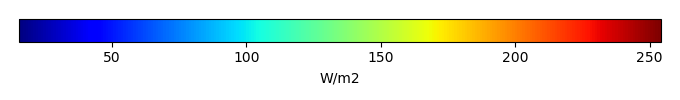MODEL MEANBIAS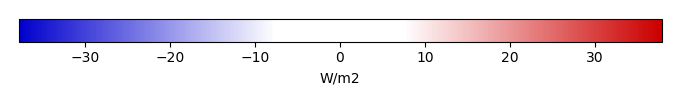BIAS SCORERMSE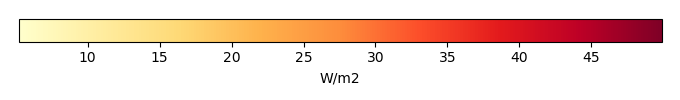RMSE SCOREBENCHMARK INTERANNUAL VARIABILITY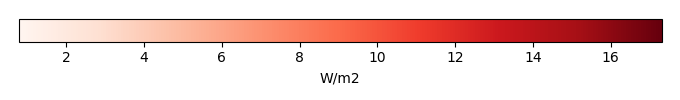MODEL INTERANNUAL VARIABILITYINTERANNUAL VARIABILITY SCOREBENCHMARK MAX MONTHMODEL MAX MONTHDIFFERENCE IN MAX MONTH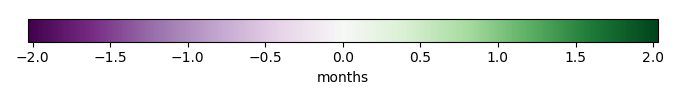SEASONAL CYCLE SCORESPATIAL TAYLOR DIAGRAMMODEL COLORS# Spatially integrated regional mean

MODEL COLORSREGIONAL MEANANNUAL CYCLEMONTHLY ANOMALYANNUAL CYCLE# All Models

BenchmarkCLM4CLM4.5CLM5# Data Information

creation_date: Thu May 8 23:03:44 PDT 2014

source_file: This product is generated from monthly 1 degree CERES EBAF Radiation observations